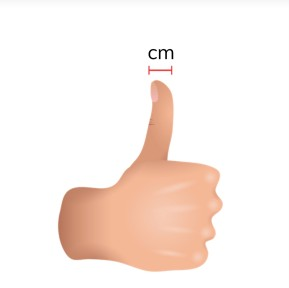Understanding what a centimeter is

# Understanding what a centimeter is

Students learn what a centimeter is.8,000 schools use Gynzy92,000 teachers use Gynzy1,600,000 students use Gynzy

## General

Students learn what a centimeter is and can indicate what objects are about a centimeter.

## Common core standard(s)

CCSS.Math.Content.2.MD.A.1

## Learning objective

Students will be able to indicate what a centimeter is and about how long a centimeter is.

## Introduction

You will play bingo together with the students. Everyone writes down nine numbers from 70 to 100. You name a number and students have to cross off the number on their card. You call out different numbers until someone has bingo.

## Instruction

Explain what a centimeter is. State that your thumb is approximately one centimeter wide. Centimeter is abbreviated as cm. Take a ruler and point to what a centimeter is. You may have students measure the width of their thumb to demonstrate that this is about a centimeter. Next you set several objects on a table, of which some are about one centimeter and some are larger. Have the students indicate which of the objects are about one centimeter. Then they must find other objects in the classroom that are about a centimeter. Afterwards, you show an image on the interactive whiteboard. Explain that a number of objects in the image are about one centimeter in real life. Have the students find these objects and drag them to the basket. Remind the students that they can compare the objects to the width of their thumb. If it is about the same width, then the object is one centimeter. Afterwards you show an image and the students indicate whether it is one, ten, or one hundred centimeters.

To check whether the students understand what a centimeter is, you can ask the following questions:
- How is centimeter abbreviated?
- What is about one centimeter?

## Quiz

First the students state whether the object is larger or smaller than one centimeter in real life. Then they must drag the images to the correct box. Finally, they must state how a centimeter is abbreviated.

## Closing

You discuss again with the students that it is useful to understand what a centimeter is, in order to be able to determine the length of an object. You check if the students understand what a centimeter is by having them indicate which objects on the interactive whiteboard are larger or smaller than one centimeter. These objects can be crossed off. Two objects remain that are about one centimeter.

## Teaching tips

When students have difficulty with understanding what a centimeter is, you can repeat that the width of your thumb is approximately one centimeter. You may show objects that are approximately one centimeter.

## Instruction materials

A ruler, examples of a centimeter (think of a thumbtack, a pencil sharpener and a dice) and objects larger than one centimeter (think of a ball, a book and a computer mouse).

### The online teaching platform for interactive whiteboards and displays in schools

• Save time building lessons

• Manage the classroom more efficiently

• Increase student engagement